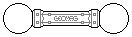Web textodigital.com
The dodecahedron made of dodecahedra
Karl Horton proposed the constructions below in this post published at the Geomag forum.
A regular dodecahedron to scale of about 3.618 can be built using 20 others: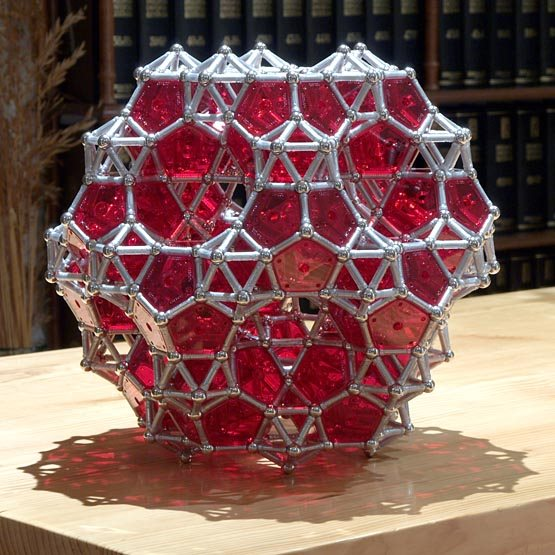The dodecahedron made of dodecahedra A, view 1 1510 pieces: 340 balls, 930 rods, 240 pentagons (8.02 kg)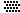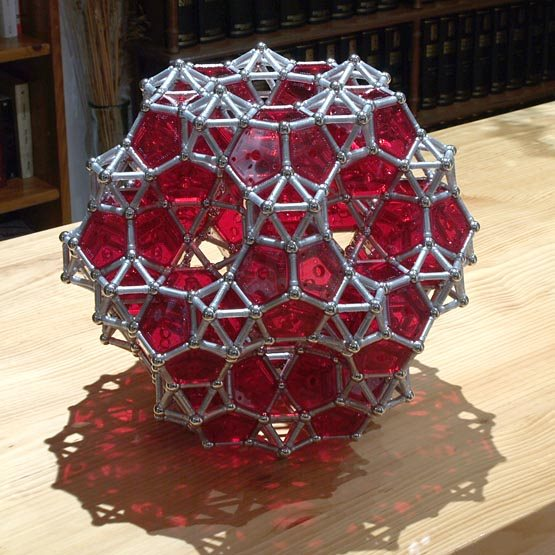The dodecahedron made of dodecahedra A, view 2
Each dodecahedron shares an edge with the three adjacent ones, and each joint is reinforced with a metabidiminished icosahedron, that is, a regular icosahedron with two adjacent pentagonal pyramids removed (see MathWorld); it can be also thought of as a normal icosahedron with two of its pyramids penetrating the adjacent dodecahedra: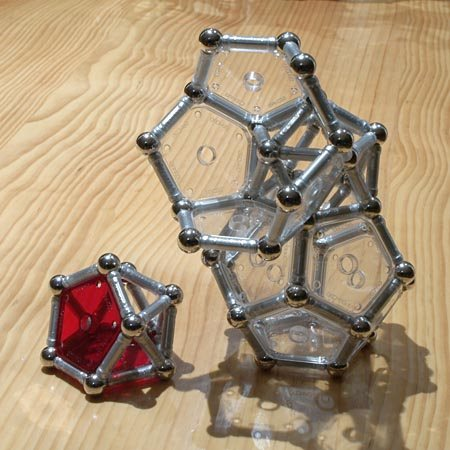Metabidiminished icosahedron and normal icosahedron
Each face of the big dodecahedron has a hole in the shape of a pentagonal rotunda, that is, a half icosidodecahedron: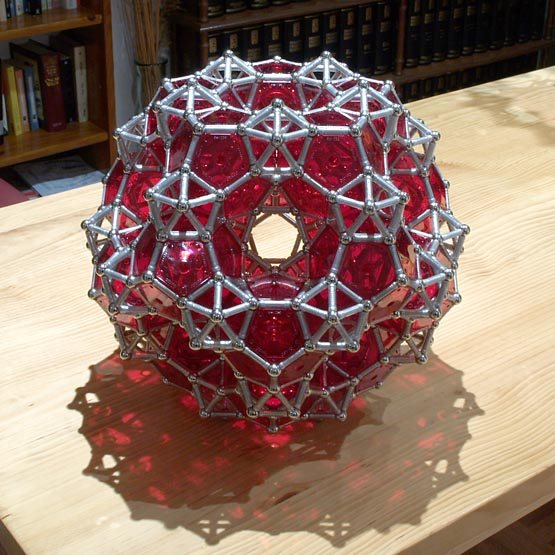The dodecahedron made of dodecahedra A, view 3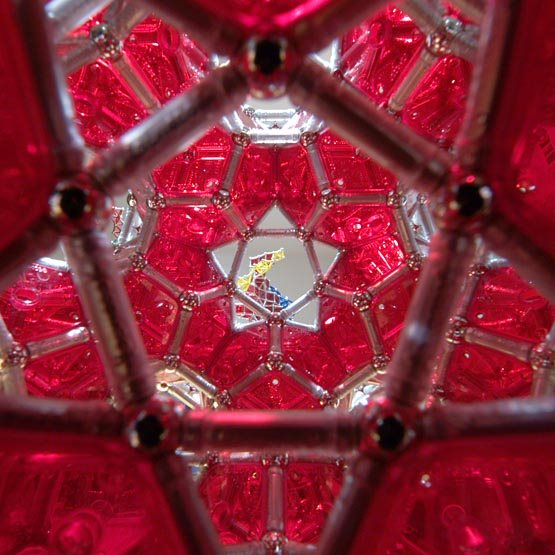The dodecahedron made of dodecahedra A, inside view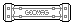If the metabidiminished icosahedron is elongated by using squares in place of the edges joining both pentagons and the opposite one, and filling the resulting holes with triangles, we get the right-hand object in this picture: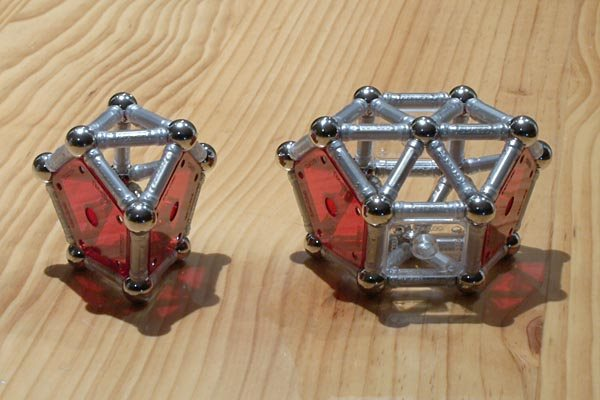Elongated metabidiminished icosahedron
When using this object to join the dodecahedra, the big dodecahedron becomes one of scale 4.618, the face holes become larger and the whole construction turns out much more pleasing: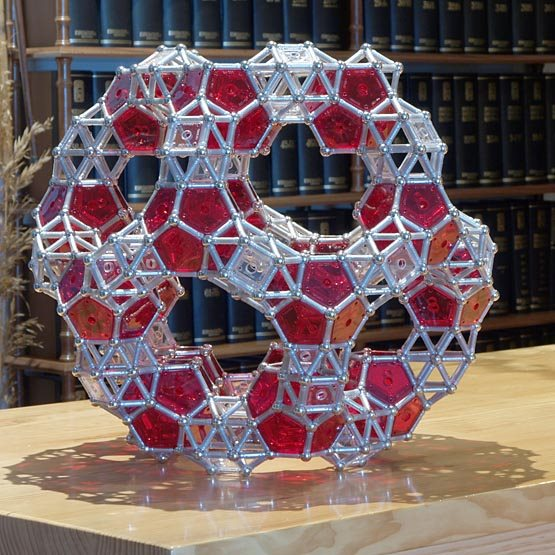The dodecahedron made of dodecahedra B, view 1 2260 pieces: 580 balls, 1380 rods, 240 pentagons, 60 squares (12.30 kg)The dodecahedron made of dodecahedra B, view 2The dodecahedron made of dodecahedra B, view 3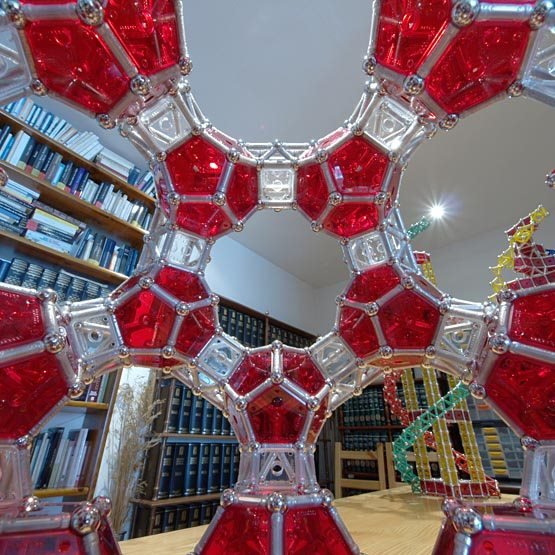The dodecahedron made of dodecahedra B, inside view